Need help? Email help@shout.education

# Theories of Acids and Bases

This page describes the Arrhenius, Bronsted-Lowry, and Lewis theories of acids and bases, and explains the relationships between them. It also explains the concept of a conjugate pair – an acid and its conjugate base, or a base and its conjugate acid.

Note: Current UK A-level syllabuses concentrate on the Bronsted-Lowry theory, but you should also be aware of Lewis acids and bases. The Arrhenius theory is of historical interest only, and you are unlikely to need it unless you are doing some work on the development of ideas in chemistry.

This page has also been translated into Portuguese by Artur Weber for https://www.homeyou.com/~edu/.

## The Arrhenius Theory of Acids and Bases

### The Theory

• Acids are substances which produce hydrogen ions in solution.
• Bases are substances which produce hydroxide ions in solution.

Neutralisation happens because hydrogen ions and hydroxide ions react to produce water.

\text{H}^+_{(aq)} + {}^-\text{OH}_{(aq)} \longrightarrow \text{H}_2\text{O}_{(l)}

### Limitations of the Theory

Hydrochloric acid is neutralised by both sodium hydroxide solution and ammonia solution. In both cases, you get a colourless solution which you can crystallise to get a white salt – either sodium chloride or ammonium chloride.

These are clearly very similar reactions. The full equations are:

\begin{aligned} \text{NaOH}_{(aq)} + \text{HCl}_{(aq)} &\longrightarrow \text{NaCl}_{(aq)} + \text{H}_2\text{O}_{(l)} \\ \\ \text{NH}_{3(aq)} + \text{HCl}_{(aq)} &\longrightarrow \text{NH}_4\text{Cl}_{(aq)} \end{aligned}

In the sodium hydroxide case, hydrogen ions from the acid are reacting with hydroxide ions from the sodium hydroxide – in line with the Arrhenius theory.

However, in the ammonia case, there don't appear to be any hydroxide ions!

But if you look at the equations carefully, the ammonia is in solution – NH3(aq). Ammonia reacts with water like this:

\text{NH}_{3(aq)} + \text{H}_2\text{O}_{(l)} \xrightleftharpoons{} {\text{NH}_4}^+_{(aq)} + {}^-\text{OH}_{(aq)}

This is a reversible reaction, and in a typical dilute ammonia solution, about 99% of the ammonia remains as ammonia molecules. Nevertheless, there are hydroxide ions there and those react with hydrogen ions in just the same way as hydroxide ions from sodium hydroxide.

So you can just about justify ammonia as being a base on the Arrhenius definition – it does produce hydroxide ions in solution. But most of the reaction is going to be a direct reaction between ammonia molecules and hydrogen ions – which doesn't fit the Arrhenius definition.

This same reaction also happens between ammonia gas and hydrogen chloride gas.

\text{NH}_{3(g)} + \text{HCl}_{(g)} \longrightarrow \text{NH}_4\text{Cl}_{(s)}

In this case, there aren't any hydrogen ions or hydroxide ions in solution – because there isn't any solution. The Arrhenius theory wouldn't count this as an acid-base reaction, despite the fact that it is producing the same product as when the two substances were in solution. That's silly!

## The Bronsted-Lowry Theory of Acids and Bases

### The Theory

• An acid is a proton (hydrogen ion) donor.
• A base is a proton (hydrogen ion) acceptor.

### The Relationship Between the Bronsted-Lowry Theory and the Arrhenius Theory

The Bronsted-Lowry theory doesn't go against the Arrhenius theory in any way – it just adds to it.

Hydroxide ions are still bases because they accept hydrogen ions from acids and form water.

An acid produces hydrogen ions in solution because it reacts with the water molecules by giving a proton to them.

When hydrogen chloride gas dissolves in water to produce hydrochloric acid, the hydrogen chloride molecule gives a proton (a hydrogen ion) to a water molecule. A co-ordinate (dative covalent) bond is formed between one of the lone pairs on the oxygen and the hydrogen from the HCl. Hydroxonium ions, H3O+, are produced.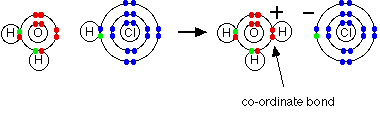\text{H}_2\text{O} + \text{HCl} \longrightarrow \text{H}_3\text{O}^+ + \text{Cl}^-

Note: If you aren't sure about co-ordinate bonding you should follow this link. Co-ordinate bonds will be mentioned several times over the course of the rest of this page.

When an acid in solution reacts with a base, what is actually functioning as the acid is the hydroxonium ion. For example, a proton is transferred from a hydroxonium ion to a hydroxide ion to make water.

\text{H}_3\text{O}^+_{(aq)} + {}^-\text{OH}_{(aq)} \longrightarrow 2\text{H}_2\text{O}_{(l)}

Showing the electrons, but leaving out the inner ones: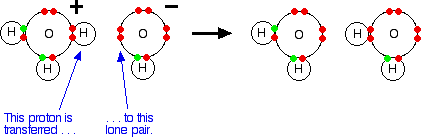It is important to realise that whenever you talk about hydrogen ions in solution, H+(aq), what you are actually talking about are hydroxonium ions.

#### The hydrogen chloride / ammonia problem

This is no longer a problem using the Bronsted-Lowry theory. Whether you are talking about the reaction in solution or in the gas state, ammonia is a base because it accepts a proton (a hydrogen ion). The hydrogen becomes attached to the lone pair on the nitrogen of the ammonia via a co-ordinate bond.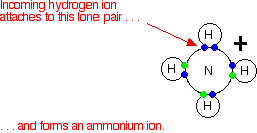If it is in solution, the ammonia accepts a proton from a hydroxonium ion:

\text{NH}_{3(aq)} + \text{H}_3\text{O}^+_{(aq)} \longrightarrow {\text{NH}_4}^+_{(aq)} + \text{H}_2\text{O}_{(l)}

If the reaction is happening in the gas state, the ammonia accepts a proton directly from the hydrogen chloride:

\text{NH}_{3(g)} + \text{HCl}_{(g)} \longrightarrow {\text{NH}_4}^+\text{Cl}^-_{(s)}

Either way, the ammonia acts as a base by accepting a hydrogen ion from an acid.

### Conjugate Pairs

When hydrogen chloride dissolves in water, almost 100% of it reacts with the water to produce hydroxonium ions and chloride ions. Hydrogen chloride is a strong acid, and we tend to write this as a one-way reaction:

\text{H}_2\text{O} + \text{HCl} \longrightarrow \text{H}_3\text{O}^+ + \text{Cl}^-

Note: I am deliberately missing state symbols off this and the next equation in order to concentrate on the bits that matter.

You will find more about strong and weak acids on another page in this section.

In fact, the reaction between HCl and water is reversible, but only to a very minor extent. In order to generalise, consider an acid HA, and think of the reaction as being reversible.

\text{HA} + \text{H}_2\text{O} \xrightleftharpoons{} \text{H}_3\text{O}^+ + \text{A}^-

• The HA is an acid because it is donating a proton (hydrogen ion) to the water.
• The water is a base because it is accepting a proton from the HA.

But there is also a back reaction between the hydroxonium ion and the A- ion:

• The H3O+ is an acid because it is donating a proton (hydrogen ion) to the A- ion.
• The A- ion is a base because it is accepting a proton from the H3O+.

The reversible reaction contains two acids and two bases. We think of them in pairs, called conjugate pairs.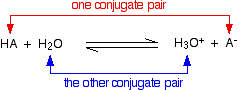When the acid, HA, loses a proton it forms a base, A-. When the base, A-, accepts a proton back again, it obviously refoms the acid, HA. These two are a conjugate pair.

Members of a conjugate pair differ from each other by the presence or absence of the transferable hydrogen ion.

If you are thinking about HA as the acid, then A- is its conjugate base.

If you are thinking about A- as the base, then HA is its conjugate acid.

The water and the hydroxonium ion are also a conjugate pair. Thinking of the water as a base, the hydroxonium ion is its conjugate acid because it has the extra hydrogen ion which it can give away again.

Thinking about the hydroxonium ion as an acid, then water is its conjugate base. The water can accept a hydrogen ion back again to reform the hydroxonium ion.

#### A second example of conjugate pairs

This is the reaction between ammonia and water that we looked at earlier:Think first about the forward reaction. Ammonia is a base because it is accepting hydrogen ions from the water. The ammonium ion is its conjugate acid – it can release that hydrogen ion again to reform the ammonia.

The water is acting as an acid, and its conjugate base is the hydroxide ion. The hydroxide ion can accept a hydrogen ion to reform the water.

Looking at it from the other side, the ammonium ion is an acid, and ammonia is its conjugate base. The hydroxide ion is a base and water is its conjugate acid.

### Amphoteric Substances

You may possibly have noticed (although probably not!) that in one of the last two examples, water was acting as a base, whereas in the other one it was acting as an acid.

A substance which can act as either an acid or a base is described as being amphoteric.

\text{H}_3\text{O} \xleftarrow[\text{\color{#467abf}{and is acting as a base}}]{\text{\color{#467abf}{water accepts a proton}}} \text{H}_2\text{O} \xrightarrow[\text{\color{#00CC99}{and is acting as an acid}}]{\text{\color{#00CC99}{water loses a proton}}} {}^-\text{OH}

Note: You might also come across the term amphiprotic in this context. The two words are related and easily confused.

An amphiprotic substance is one which can both donate hydrogen ions (protons) and also accept them. Water is a good example of such a compound. The water acts as both an acid (donating hydrogen ions) and as a base (by accepting them). The "protic" part of the word refers to the hydrogen ions (protons) either being donated or accepted. Other examples of amphiprotic compounds are amino acids, and ions like HSO4- (which can lose a hydrogen ion to form sulfate ions or accept one to form sulfuric acid).

But as well as being amphiprotic, these compounds are also amphoteric. Amphoteric means that they have reactions as both acids and bases. So what is the difference between the two terms?

All amphiprotic substances are also amphoteric – but the reverse isn't true. There are amphoteric substances which don't either donate or accept hydrogen ions when they act as acids or bases. There is a whole new definition of acid-base behaviour that you are just about to meet (the Lewis theory) which doesn't necessarily involve hydrogen ions at all.

A Lewis acid is an electron pair acceptor; a Lewis base is an electron pair donor (see below).

Some metal oxides (like aluminium oxide) are amphoteric – they react both as acids and bases. For example, they react as bases because the oxide ions accept hydrogen ions to make water. That's not a problem as far as the definition of amphiprotic is concerned – but the reaction as an acid is. The aluminium oxide doesn't contain any hydrogen ions to donate! But aluminium oxide reacts with bases like sodium hydroxide solution to form complex aluminate ions.

You can think of lone pairs on hydroxide ions as forming dative covalent (coordinate) bonds with empty orbitals in the aluminium ions. The aluminium ions are accepting lone pairs (acting as a Lewis acid). So aluminium oxide can act as both an acid and a base – and so is amphoteric. But it isn't amphiprotic because both of the acid reaction and the base reaction don't involve hydrogen ions.

## The Lewis Theory of Acids and Bases

This theory extends well beyond the things you normally think of as acids and bases.

### The Theory

• An acid is an electron pair acceptor.
• A base is an electron pair donor.

### The Relationship Between the Lewis Theory and the Bronsted-Lowry Theory

#### Lewis bases

It is easiest to see the relationship by looking at exactly what Bronsted-Lowry bases do when they accept hydrogen ions. Three Bronsted-Lowry bases we've looked at are hydroxide ions, ammonia and water, and they are typical of all the rest.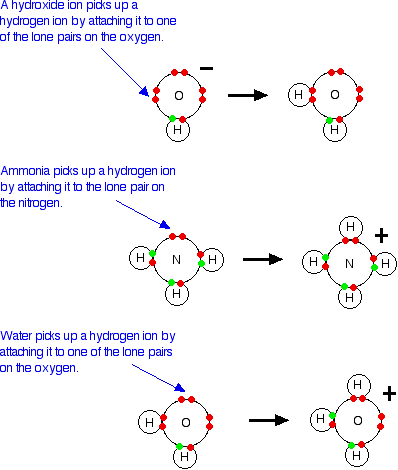The Bronsted-Lowry theory says that they are acting as bases because they are combining with hydrogen ions. The reason they are combining with hydrogen ions is that they have lone pairs of electrons – which is what the Lewis theory says. The two are entirely consistent.

So how does this extend the concept of a base? At the moment it doesn't – it just looks at it from a different angle.

But what about other similar reactions of ammonia or water, for example? On the Lewis theory, any reaction in which the ammonia or water used their lone pairs of electrons to form a co-ordinate bond would be counted as them acting as a base.

Here is a reaction which you will find talked about on the page dealing with co-ordinate bonding. Ammonia reacts with BF3 by using its lone pair to form a co-ordinate bond with the empty orbital on the boron.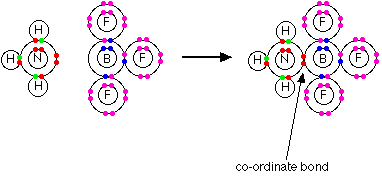As far as the ammonia is concerned, it is behaving exactly the same as when it reacts with a hydrogen ion – it is using its lone pair to form a co-ordinate bond. If you are going to describe it as a base in one case, it makes sense to describe it as one in the other case as well.

Note: If you haven't already read the page about co-ordinate bonding you should do so now. You will find an important example of water acting as a Lewis base as well as this example – although the term Lewis base isn't used on that page.

#### Lewis acids

Lewis acids are electron pair acceptors. In the above example, the BF3 is acting as the Lewis acid by accepting the nitrogen's lone pair. On the Bronsted-Lowry theory, the BF3 has nothing remotely acidic about it.

This is an extension of the term acid well beyond any common use.

What about more obviously acid-base reactions – like, for example, the reaction between ammonia and hydrogen chloride gas?

\text{NH}_{3(g)} + \text{HCl}_{(g)} \longrightarrow {\text{NH}_4}^+_{(s)} + \text{Cl}^-_{(s)}

What exactly is accepting the lone pair of electrons on the nitrogen. Textbooks often write this as if the ammonia is donating its lone pair to a hydrogen ion – a simple proton with no electrons around it.

That is misleading! You don't usually get free hydrogen ions in chemical systems. They are so reactive that they are always attached to something else. There aren't any uncombined hydrogen ions in HCl.

There isn't an empty orbital anywhere on the HCl which can accept a pair of electrons. Why, then, is the HCl a Lewis acid?

Chlorine is more electronegative than hydrogen, and that means that the hydrogen chloride will be a polar molecule. The electrons in the hydrogen-chlorine bond will be attracted towards the chlorine end, leaving the hydrogen slightly positive and the chlorine slightly negative.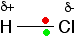Note: If you aren't sure about electronegativity and bond polarity it might be useful to follow this link.

The lone pair on the nitrogen of an ammonia molecule is attracted to the slightly positive hydrogen atom in the HCl. As it approaches it, the electrons in the hydrogen-chlorine bond are repelled still further towards the chlorine.

Eventually, a co-ordinate bond is formed between the nitrogen and the hydrogen, and the chlorine breaks away as a chloride ion.

This is best shown using the "curly arrow" notation commonly used in organic reaction mechanisms.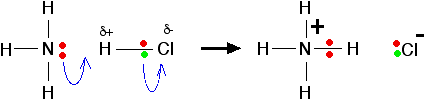Note: If you aren't happy about the use of curly arrows to show movements of electron pairs, you should follow this link.

The whole HCl molecule is acting as a Lewis acid. It is accepting a pair of electrons from the ammonia, and in the process it breaks up. Lewis acids don't necessarily have to have an existing empty orbital.

### A Final Comment on Lewis Acids and Bases

If you are a UK A-level student, you might occasionally come across the terms Lewis acid and Lewis base in textbooks or other sources. All you need to remember is:

• A Lewis acid is an electron pair acceptor.
• A Lewis base is an electron pair donor.

Note: Remember this by thinking of ammonia acting as a base. Most people at this level are familiar with the reactive lone pair on the nitrogen accepting hydrogen ions. Ammonia is basic because of its lone pair. That means that bases must have lone pairs to donate. Acids are the opposite.

For all general purposes, stick with the Bronsted-Lowry theory.